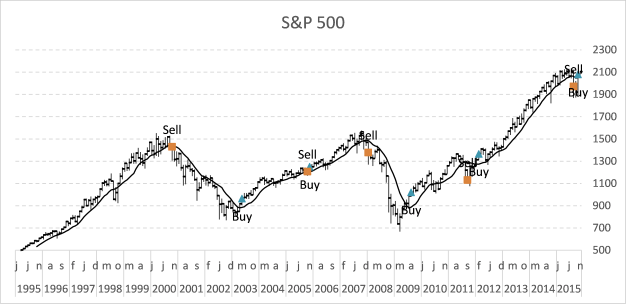Author: Oscar Cronquist Article last updated on May 24, 2021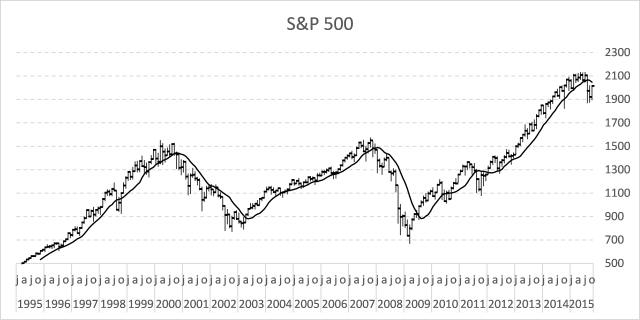In my previous post, I described how to build a dynamic stock chart that lets you easily adjust the date range and change index/company. Price data is quickly and automatically fetched from yahoo finance.

This post shows you how to add tools to the charts so you can quickly identify the trend for a stock or index. The stock market often trends for many months up or down and a moving average smooths out price data.

The examples shown in this post are based on S&P 500 index and larger trends. Charts show price data on a monthly scale and the date range is from 1995 to the present time.

This Excel stock chart has a 10-month moving average. It can be calculated using the AVERAGE function on an excel worksheet.

## 1. Calculate moving average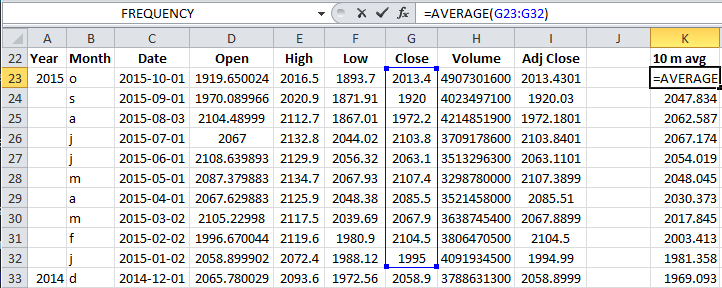Formula in cell K23:

=AVERAGE(G24:G33)

This function calculates the average from 10 latest closing prices. Copy cell K23 and paste to cells below as far as needed.

## 2. How to add a moving average to an Excel stock chart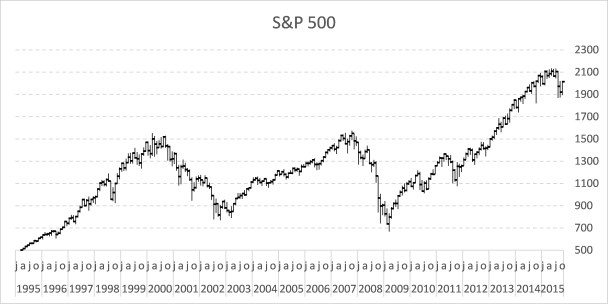1. Press with right mouse button on on chart
2. Press with mouse on "Select Data..."
3. Press with left mouse button on "Add" button
4. Select cell range \$K\$23:\$K\$2725. Press with left mouse button on OK button
6. Go to tab "Layout" on the ribbon.
7. Select "Series 4"8. Press with left mouse button on "Format Selection" button, see picture above.
9. Select "Secondary Axis"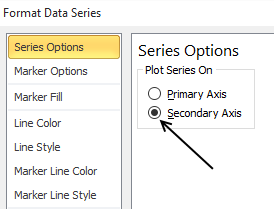10. Go to "Line Color"
11. Select "Solid Line"
12. Pick a color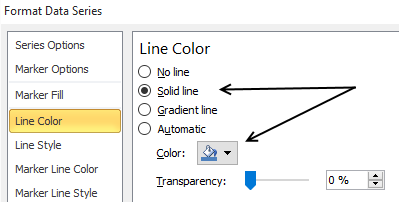The chart now looks like this: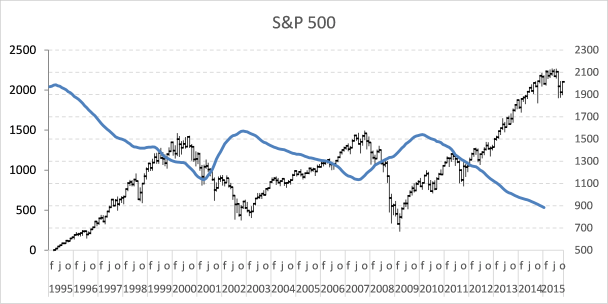The data on the secondary axis has to be reversed.

1. Select line on chart
2. Go to tab "Layout" on the ribbon
3. Press with left mouse button on "Axis" button and then "Secondary horizontal axis" and finally press with left mouse button on "Show right to left axis"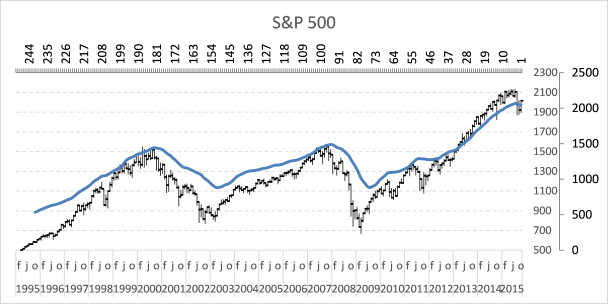4. Select and delete the horizontal axis above the chart
5. Go to tab "Layout" on the ribbon
6. Press with left mouse button on "Axis" button and "Secondary Vertical Axis" and finally "None"The chart looks like this: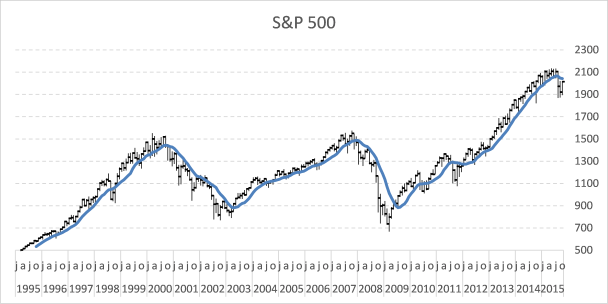A moving average indicates if a market is about to go up or down in the long term.

## 3. Plot moving average turning points

Let's start with calculating when the moving average changes from moving down to up.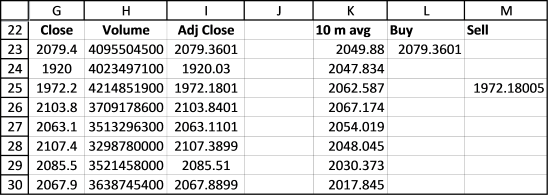Buy formula in cell L23:

=IF((K23>K24)*(K24<K25),G23,"")

If the value in cell K23 is larger than cell K24 AND cell K24 is smaller than K25 THEN return the closing price.  If not return nothing.

The next formula calculates when the moving average changes from going up to going down.

Sell formula in cell M23:

=IF((K23<K24)*(K24>K25),G23,"")

Copy cell range L23:M23 and paste to cells below as far as needed. It is now time to plot these moving average turning points.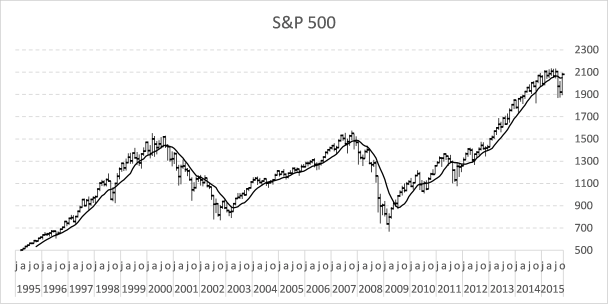1. Press with right mouse button on on chart
2. Press with left mouse button on "Select Data..."
3. Press with left mouse button on "Add" button
4. Select cell range \$L\$23:\$L\$273
5. Press with left mouse button on OK button
6. Press with left mouse button on "Add" button again
7. Select cell range \$M\$23:\$M\$273
8. Press with left mouse button on OK button
9. Press with left mouse button on OK button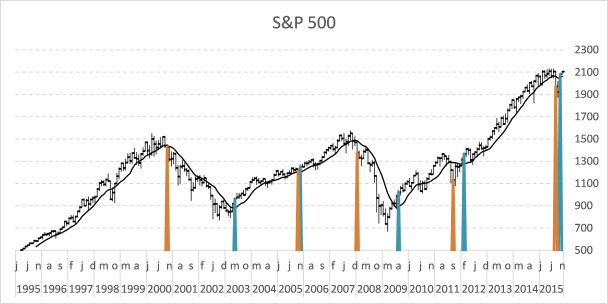Here is how to remove lines shown above and use markers instead.

1. Go to tab "Layout"
2. Select Series 5
3. Press with left mouse button on "Format Selection"
4. Select "Marker Options"
5. Select "Built-in"
6. Pick a type
7. Go to "Line Color"
8. Select "No Line"
9. Select "Series 6" on tab "Layout" on the ribbon
10. Select "Marker Options"
11. Select "Built-in"
12. Pick a type
13. Go to "Line Color"
14. Select "No Line"## 4. How to extract the moving average turning dates using Excel array formulas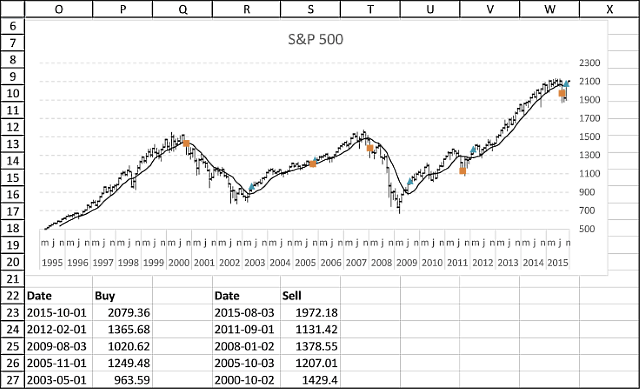Array formula (Buy) in cell P23:

=IFERROR(INDEX(\$G\$23:\$G\$262, SMALL(IF((\$K\$23:\$K\$262>\$K\$24:\$K\$263)*(\$K\$24:\$K\$263<\$K\$25:\$K\$264), MATCH(ROW(\$G\$23:\$G\$262), ROW(\$G\$23:\$G\$262)), ""), ROWS(\$A\$1:A1))), "")

Formula (Buy Date) in cell O23:

=IFERROR(INDEX(\$C\$23:\$C\$262,MATCH(P23,\$G\$23:\$G\$262,0)),"")

Array formula (Sell) in cell S23:

=IFERROR(INDEX(\$G\$23:\$G\$262, SMALL(IF((\$K\$23:\$K\$262<\$K\$24:\$K\$263)*(\$K\$24:\$K\$263>\$K\$25:\$K\$264), MATCH(ROW(\$G\$23:\$G\$262), ROW(\$G\$23:\$G\$262)), ""), ROWS(\$A\$1:A1))), "")

Formula (Sell Date) in cell R23:

=IFERROR(INDEX(\$C\$23:\$C\$262,MATCH(S23,\$G\$23:\$G\$262,0)),"")

### 4.1 How to enter an array formula

1. Select cell P23
2. Copy array formula
3. Paste array formula in the formula bar
4. Press and hold CTRL + SHIFT simultaneously
5. Press Enter once
6. Release all keys

If you did the above instructions correctly, the formula begins and ends with a curly bracket, like this {=formula}. Don´t enter these characters yourself.

Make sure you enter array formulas in cell P23 and S23. Then copy cell P23 and paste to cells below as far as needed. Repeat with cell S23, R23 and O23.

### 4.2 Explaining formula in cell P23

#### Step 1 - Check if each value in \$K\$23:\$K\$262 is larger than the next cell value below

The less than sign checks if the numerical value in a cell is less than the number in the next cell below.

\$K\$23:\$K\$262<\$K\$24:\$K\$263

The less than sign is a logical operator and the result is a boolean value, TRUE or FALSE.

#### Step 2 - Check if each value in \$K\$24:\$K\$263 is larger than the next cell value below

The larger than sign checks if the numerical value in a cell is larger than the number in the next cell below.

\$K\$24:\$K\$263>\$K\$25:\$K\$264

The larger than sign is also a logical operator and the result is a boolean value, TRUE or FALSE.

#### Step 3 - Multiply arrays

When we multiply arrays using the asterisk sign we apply AND-logic meaning TRUE is returned only if TRUE is present in the same position in both arrays.

TRUE * TRUE = TRUE

TRUE * FALSE = FALSE

FALSE * TRUE = FALSE

FALSE * FALSE = FALSE

(\$K\$23:\$K\$262>\$K\$24:\$K\$263)*(\$K\$24:\$K\$263<\$K\$25:\$K\$264)

The numerical equivalent is returned when two boolean values are multiplied.

TRUE = 1

FALSE = 0 (zero)

#### Step 4 - Create sequence from 1 to n

The ROW function returns a number representing the row based on a cell reference, for example, ROW(A1) returns 1.

This works also if we use a cell range as a cell reference, however, the formula is now an array formula. It calculates an array of values.

MATCH(ROW(\$G\$23:\$G\$262), ROW(\$G\$23:\$G\$262))

The MATCH function returns the relative position of a given value.

MATCH({23; 24; 25; ... ; 262}, {23; 24; 25; ... ; 262})

and returns {1; 2; 3; ... }.

#### Step 5 - Returns row number if logical expression is TRUE

The IF function returns one value if the logical test is TRUE and another value if the logical test is FALSE.

IF(logical_test, [value_if_true], [value_if_false])

IF((\$K\$23:\$K\$262>\$K\$24:\$K\$263)*(\$K\$24:\$K\$263<\$K\$25:\$K\$264), MATCH(ROW(\$G\$23:\$G\$262), ROW(\$G\$23:\$G\$262)), "")

#### Step 6 - Extract the k-th smallest row number

The SMALL function returns the k-th smallest value from a group of numbers.

SMALL(arrayk)

The ROWS function returns the number of rows based on a cell reference.

ROWS(array)

SMALL(IF((\$K\$23:\$K\$262>\$K\$24:\$K\$263)*(\$K\$24:\$K\$263<\$K\$25:\$K\$264), MATCH(ROW(\$G\$23:\$G\$262), ROW(\$G\$23:\$G\$262)), ""), ROWS(\$A\$1:A1)))

#### Step 7 - Get corresponding value from column G

The INDEX function returns a value from a cell range, you specify which value based on a row and column number.

INDEX(array[row_num][column_num])

INDEX(\$G\$23:\$G\$262, SMALL(IF((\$K\$23:\$K\$262<\$K\$24:\$K\$263)*(\$K\$24:\$K\$263>\$K\$25:\$K\$264), MATCH(ROW(\$G\$23:\$G\$262), ROW(\$G\$23:\$G\$262)), ""), ROWS(\$A\$1:A1)))

#### Step 8 - Handle errors

The IFERROR function lets you catch most errors in Excel formulas.

IFERROR(valuevalue_if_error)

IFERROR(INDEX(\$G\$23:\$G\$262, SMALL(IF((\$K\$23:\$K\$262>\$K\$24:\$K\$263)*(\$K\$24:\$K\$263<\$K\$25:\$K\$264), MATCH(ROW(\$G\$23:\$G\$262), ROW(\$G\$23:\$G\$262)), ""), ROWS(\$A\$1:A1))), "")

This template has dynamic named ranges for all chart series. This lets you change the date range and all chart data is adjusted automatically. I have not described how they work in this post, if you are curious

The template also contains a small custom function to fetch stock data from yahoo finance.

### Get the Excel fileFollow-stock-market-trends-moving-avgv2.xlsm

Tip! - Add data labels